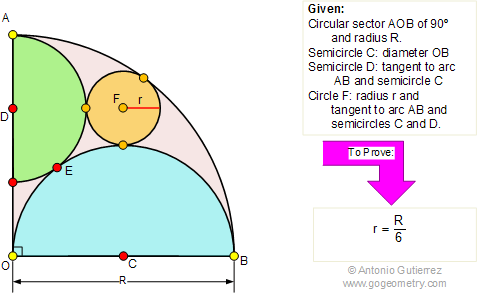Problem 285: Circular Sector 90 degrees, Semicircles, Circle, Tangent, Radius The figure shows a circular sector AOB of 90 degrees with radius R. OB is the diameter of semicircle C. Semicircle D is tangent to semicircle C and arc AB at E and A, respectively. Circle F is tangent to arc AB and semicircles D and C. If r is the radius of the circle F, prove that r = R / 6.Recent Additions